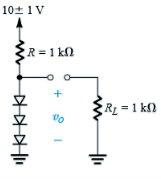Courses

# Test: Modelling The Diode Forward Characteristics

## 10 Questions MCQ Test Electronic Devices | Test: Modelling The Diode Forward Characteristics

Description
This mock test of Test: Modelling The Diode Forward Characteristics for Electrical Engineering (EE) helps you for every Electrical Engineering (EE) entrance exam. This contains 10 Multiple Choice Questions for Electrical Engineering (EE) Test: Modelling The Diode Forward Characteristics (mcq) to study with solutions a complete question bank. The solved questions answers in this Test: Modelling The Diode Forward Characteristics quiz give you a good mix of easy questions and tough questions. Electrical Engineering (EE) students definitely take this Test: Modelling The Diode Forward Characteristics exercise for a better result in the exam. You can find other Test: Modelling The Diode Forward Characteristics extra questions, long questions & short questions for Electrical Engineering (EE) on EduRev as well by searching above.
QUESTION: 1

### Which of the following is method to model a diode’s forward characteristics?

Solution:

All of the mentioned are different methods used to model a diode’s forward characteristics.

QUESTION: 2

### A voltage regulator needs to provide a constant voltage in spite of the fact that there may be

Solution:

Voltage regulators are required in both of the situations mentioned.

QUESTION: 3

### For the circuit shown below calculateCalculate the %age change in the regulated voltage caused by a change of ±10% in the input voltage. (RL is not connected to the circuit)

Solution:

Current I in the circuit = (10 – 2.1) / 1 or 7.9 mA
Incremental resistance of each diode is rd = 25mV/7.9mA at room temperature or 3.2Ω
Total resistance of the diode connection = r = 9.6Ω
The series combination of R and r acts as a voltage divider, hence
▲V0 = ±r/r+R or 9.5 mA or ± 0.5%.

QUESTION: 4Calculate the change in the voltage when RL is connected as shown

Solution:

When RL is connected it draws approx. 2.1v/1000 A current or 2.1 mA. Hence the current in the diode decreases by approximately 2.1 mA. The voltage change across the diode is thus -2.1mA * 9.6 Ω or -20mA.

QUESTION: 5

The value of the diode small-signal resistance rd at bias currents of 0.1 mA is

Solution:

Thermal voltage at room temperature is 25mV and the current is 0.1mA. rd = 25mV/0.1mA or 250 Ω.

QUESTION: 6

In many applications, a conducting diode is modeled as having a constant voltage drop, usually approximately

Solution:

Normally a silicon diode has a voltage drop of 0.7V.

QUESTION: 7

The graphical method of modeling a diode characteristics is based on

Solution:

Graphical method uses equations obtained in the exponential method and then the Answer of these equations is obtained by plotting them on a graph.

QUESTION: 8

For small-signal operation around the dc bias point, the diode is modeled by a resistance equal to the

Solution:

It is a required characteristic for small-ground operations.

QUESTION: 9

The other name for bias point is

Solution:

Quiescent point is also called base point. It is due to this name that is also called Q–point.

QUESTION: 10

In iteration method for modelling a diode the answer obtained by each subsequent iteration is

Solution:

True value is obtained by the exponential method and therefore both of the statements are true.